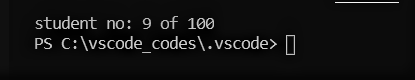Open in App
Not now

# Read a record from a File in C++ using seekg() and tellg()

• Difficulty Level : Medium
• Last Updated : 16 Dec, 2022

Given that a binary file “student.data” is already loaded in the memory of the computer with the record of 100 students, the task is to read the Kth record and perform some operations.
seekg() is a function in the iostream library (part of the standard library) that allows you to seek to an arbitrary position in a file. It is used in file handling to sets the position of the next character to be extracted from the input stream from a given file. For example :

```Input: "Hello World"
Output: World```

The tellg() function is used with input streams and returns the current “get” position of the pointer in the stream. It has no parameters and returns a value of the member type pos_type, which is an integer data type representing the current position of the get stream pointer.
Approach:
Given that there are 100 records in the binary file “student.dat” and K = 7

• Step 1: The statement fs.seekg(7*sizeof(student)) places the reading pointer to 168(->7*22) index of the file (based on ‘0’ based indexing)

• Step 2:The statement fs.read((char*)this;sizeof(student)); reads the record and now the read pointer is at the starting of 8th record. Therefore the statement fs.tellg()/sizeof(s) results to value 8 and the output of “fs.tellg()/sizeof(s)+1” is 8 + 1 = 9

• Step 3: The statement fs.seekg(0, ios::end) places the pointer to the end of the file and hence fs.tellg(0, ios::end)/sizeof(s) gives 100

Below is the implementation of the above approach:

## CPP

 `// C++ program to Read a record from a File``// using seekg() and tellg()` `#include ``using` `namespace` `std;` `class` `student {``    ``int` `id;``    ``char` `Name;` `public``:``    ``void` `display(``int` `K);``};` `void` `student::display(``int` `K)``{``    ``fstream fs;``    ``fs.open(``"student.dat"``, ios::in | ios::binary);` `    ``// using seekg(pos) method``    ``// to place pointer at 7th record``    ``fs.seekg(K * ``sizeof``(student));` `    ``// reading Kth record``    ``fs.read((``char``*)``this``, ``sizeof``(student));` `    ``// using tellg() to display current position``    ``cout << ``"Current Position: "``         ``<< ``"student no: "``         ``<< fs.tellg() / ``sizeof``(student) + 1;` `    ``// using seekg()place pointer at end of file``    ``fs.seekg(0, ios::end);` `    ``cout << ``" of "``         ``<< fs.tellg() / ``sizeof``(student)``         ``<< endl;``    ``fs.close();``}` `// Driver code``int` `main()``{` `    ``// Record number of the student to be read``    ``int` `K = 7;` `    ``student s;``    ``s.display(K);` `    ``return` `0;``}`

Output:

`Current Position: student no: 9 of 100`My Personal Notes arrow_drop_up